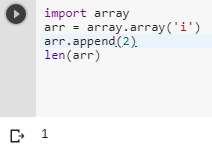# Is arr.__len__() the preferred way to get the length of an array in Python?

1 view
in Python

In Python,  is the following the only way to get the number of elements?

arr.__len__()

If so, why the strange syntax?

by (107k points)
edited by
• You can use the following way for getting the length of an array in Python

my_list = [1,2,3,4,5]

len(my_list)• So instead of arr.__len__() you can use:-

import array

arr = array.array('i')

arr.append('2')

len(arr)